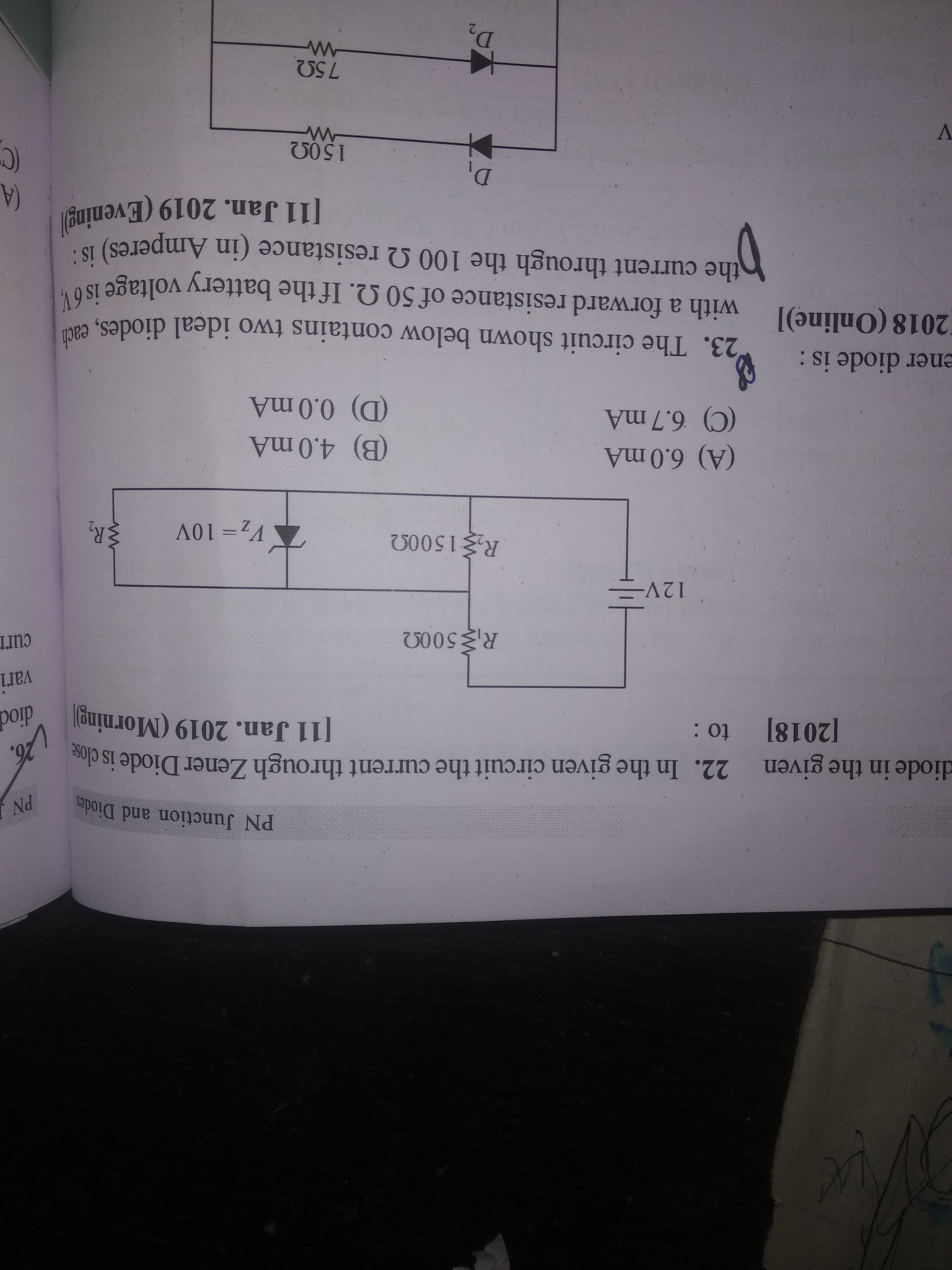# Doubt from zener diodeQ22

Look here potential across across zener diode is 7.2 which is less than 10 so no zener breakdown happen so no net current flows through zener diode . Hence D.Thanks

Can you tell how you have calculated 7.2?

I have calculated the potenial difference between R2 resisistor which is same As potenial difference between zener diode.

What to do with resistance R?

R?? R1 or R2

I mean R2 of which no value is given

Well its value be 1500. Both r2 are in parallel so use net resistance 750 and find potenial potenial difference between them which is 7.2.